## 切削速度(VC)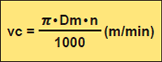*用1000去除，为将mm换算成m
VC(m/min):切削速度
Dm(mm):工件材料直径
π(3.14):圆周率
n(min-1):主轴转速

n(min-1)
Dm(mm)
vc(m/min)

### 例题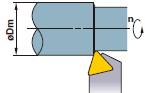### 答

π=3.14、Dm=50、n=700代入公式
vc=(π×Dm×n)÷1000=(3.14×50×700)÷1000
=110(m/min)

## 进给量(f)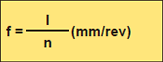f(mm/rev):每转进给量
l(mm/min):每分钟切削长度
n(min-1):主轴转速

l(mm/min)
n(min-1)
f(mm/rev)

### 例题### 答

n=500、l=120代入公式
f=l÷n=120÷500=0.24 mm/rev

## 切削时间(Tc)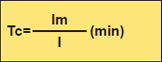Tc(min):切削时间
lm(mm):工件长度
ｌ(mm/min):每分钟的切削长度

lm(mm)
l(mm/min)
Tc(min)

### 答

l=f×n=0.2×1000=200 mm/min

Tc=lm÷l=100÷200=0.5min
0.5×60=30(sec)30秒

## 理论表面粗糙度（h）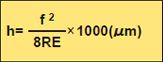h (μm):表面粗糙度
f (mm/rev):每转进给量
RE (mm):刀尖圆弧半径

f(mm/rev)
RE(mm)
h(μm）

### 例题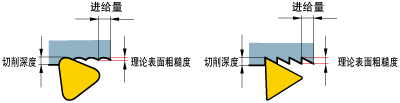### 答

h=0.22÷(8×0.8)×1000=6.25μm